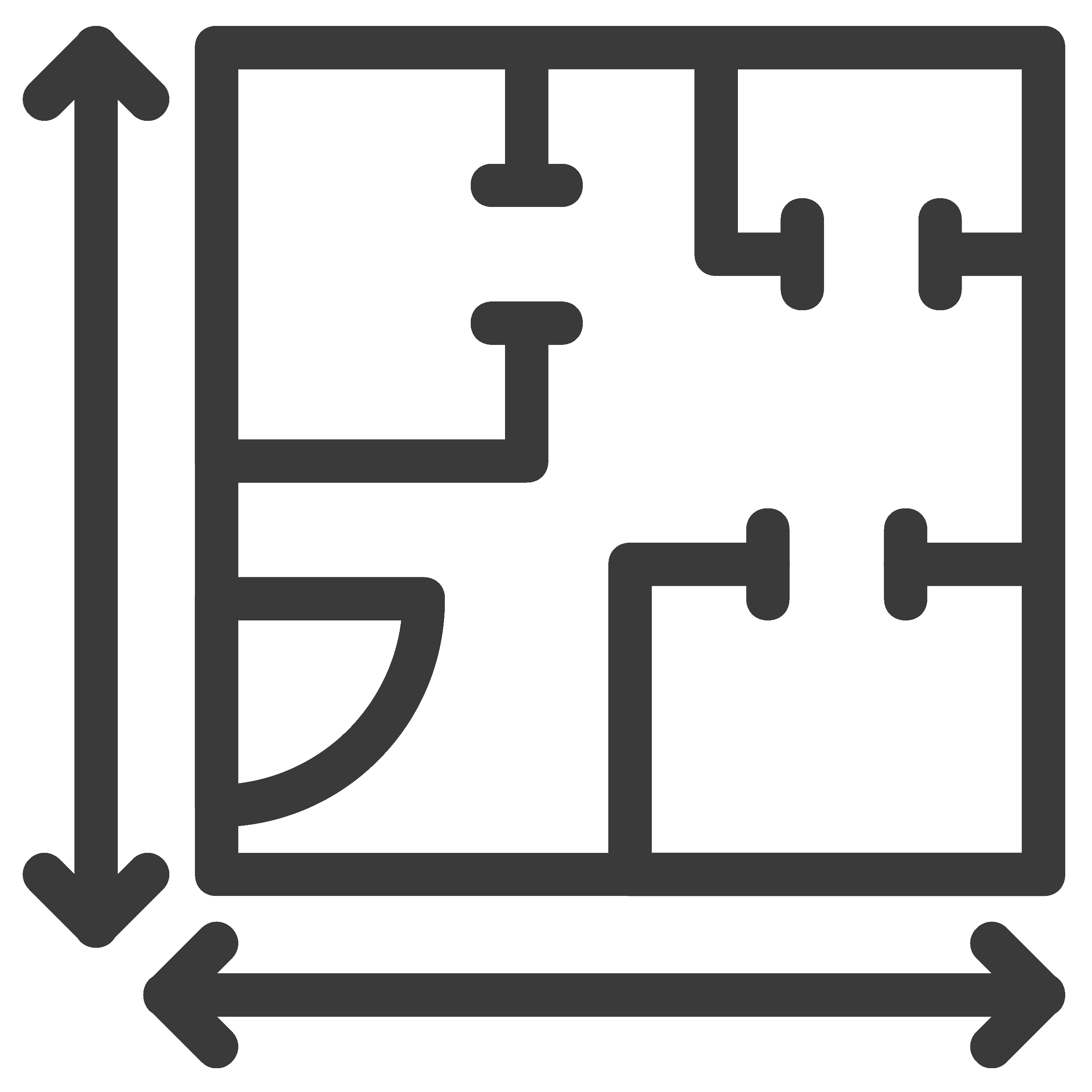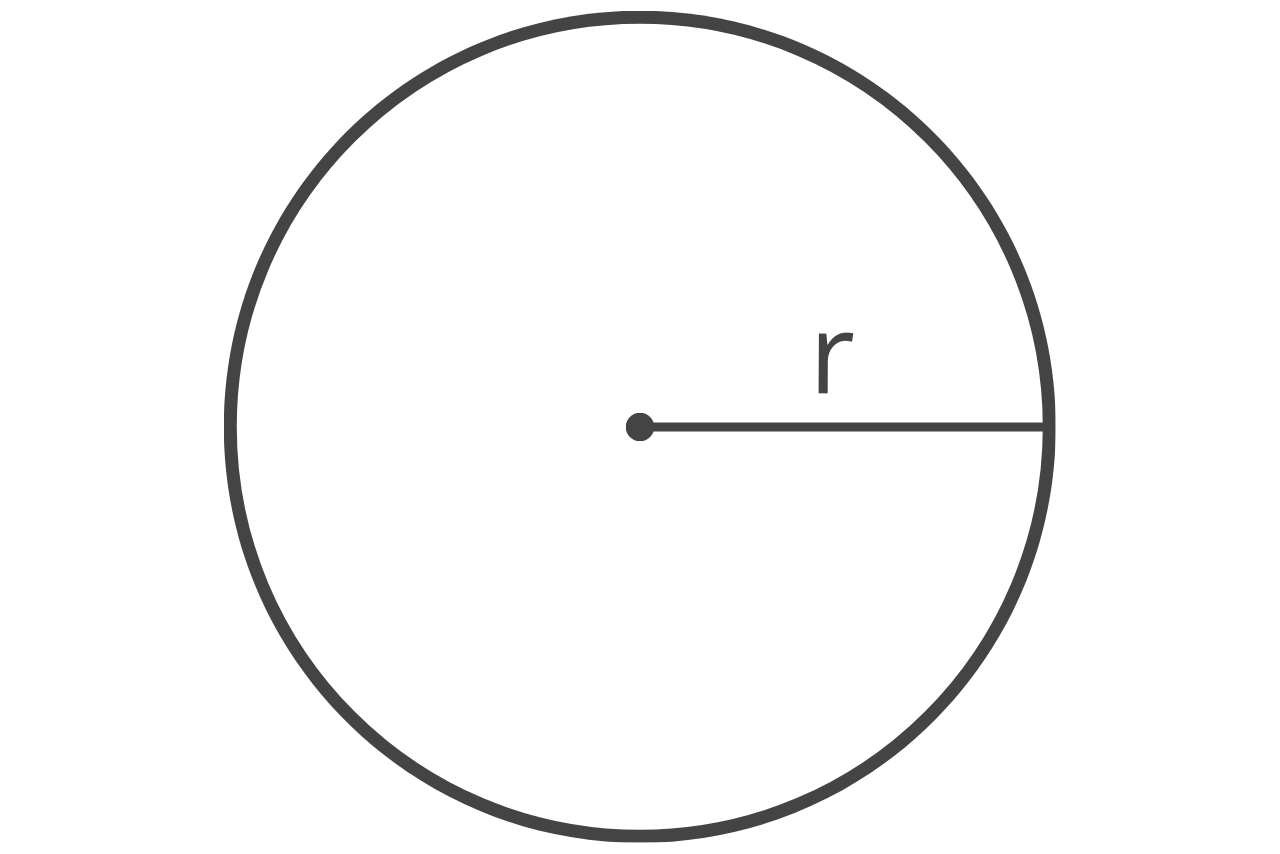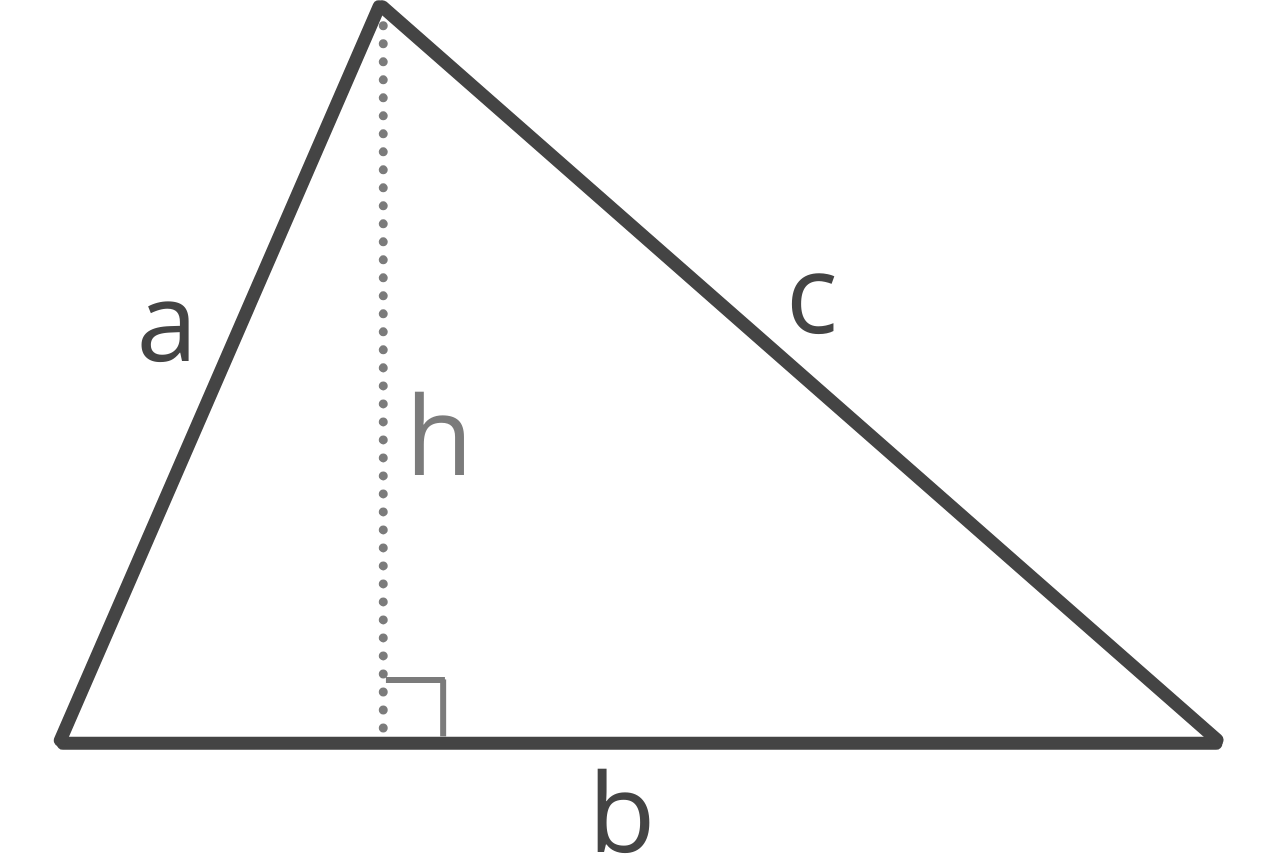# Square Footage Calculator

Find the square footage of an area by select its shape and entering the dimensions. Include the price per to estimate the cost of materials. Dimensions can be entered in inches, feet, yards, centimeters, or meters.

Select a Shape:

## Rectangle Square Footage

Optionally enter the price per square foot
\$

## Square Square Footage

Optionally enter the price per square foot
\$

## Border Square Footage

Optionally enter the price per square foot
\$

## Trapezoid Square Footage

Optionally enter the price per square foot
\$

## Parallelogram Square Footage

Optionally enter the price per square foot
\$

## Triangle Square Footage

Optionally enter the price per square foot
\$

## Circle Square Footage

Optionally enter the price per square foot
\$

## Ellipse Square Footage

Optionally enter the price per square foot
\$

## Sector Square Footage

Optionally enter the price per square foot
\$

## Regular Polygon Square Footage

Optionally enter the price per square foot
\$

\$

## How to Find Square Footage

Square footage is just an area, measured in feet, and it’s often abbreviated sq. ft. or ft2. It’s often used for construction, home remodeling, and renovation projects such as carpeting, hardwood flooring, tiling, framing, drywalling, painting, and gardening material estimates.

Find square feet just like you would find the area of a shape, by multiplying the length times the width.

Before multiplying the length and width together, convert all measurements to feet.

To calculate the square footage when measurements are in inches or another unit of measure, first convert the length and width measurements to feet, then multiply them together.Find the area of a room or space in square feet by following these easy steps:

• Measure the length and width of the area.
• Convert these measurements to feet if they aren’t already. Convert inches to feet, convert yards to feet, or convert meters to feet.
• Multiply the length in feet times the width in feet
• To estimate the cost of materials, multiply the total square footage by the price per square foot.
• If the area is not a simple shape, break it into manageable parts and calculate the area of each part separately and then add them together. For example, to measure a floor of your home, calculate the square feet of each room, then add all area measurements together to derive the total square footage.

For example, let’s find the square feet of a room that is 12′ wide by 16′ long.

area = 12′ × 16′
area = 192 sq. ft.

### How to Calculate Square Footage for Different Shapes

Use the formulas below to find the square footage for various shapes. Convert all measurements to feet before using the formulas.

#### Rectangle

sq. ft. = l × w

l = length
w = width#### Rectangular Border

sq. ft. = (l – (2 × b)) × (w – (2 × b))

l = outer length
w = outer width
b = border width#### Circle

sq. ft. = πr2

π = 3.14159265359

If you know the diameter of the circle you can find the radius by dividing the diameter in half.

Calculate square feet of a circle#### Triangle

s = 1/2(a + b + c)
sq. ft. = s(s – a)(s – b)(s – c))

a = edge a
b = edge b
c = edge cOur area calculator has even more formulas.

## Calculating the Square Feet of a Home or Residence

If you’re measuring the square footage of a home or residence, there are some special considerations that apply to determine inhabitable square footage. The livable size of a home helps determine the market value and price of a home and also helps buyers understand the size of the home.

Only rooms that are livable, with finished walls, floor, and ceiling, count toward the finished area of the home. For a room to be considered livable the space should be finished and should have heating or air conditioning as applicable. Indoor spaces count toward the square footage of a home, outdoor spaces typically do not.

To calculate the total area, measure each room in feet using a tape measure, then multiply the length and width of each room to get the square footage, then add them all together. The calculator above can help determine the square feet of each room, then simply add all of the room’s areas together. We also have great resources on how to measuring rooms and complex spaces.

To calculate the price per square foot of your home, divide the total price by the number of square feet.

price per ft2 = total price ÷ total ft2

For example, to find the price per ft2 of a home that costs \$200,000 and is 2,000 ft2 use this formula.

price per ft2 = \$200,000 ÷ 2,000 ft2
price per ft2 = \$100